NEET  >  31 Year NEET Previous Year Questions: System of Particles & Rotational Motion - 1

# 31 Year NEET Previous Year Questions: System of Particles & Rotational Motion - 1

Test Description

## 15 Questions MCQ Test Physics Class 11 | 31 Year NEET Previous Year Questions: System of Particles & Rotational Motion - 1

31 Year NEET Previous Year Questions: System of Particles & Rotational Motion - 1 for NEET 2023 is part of Physics Class 11 preparation. The 31 Year NEET Previous Year Questions: System of Particles & Rotational Motion - 1 questions and answers have been prepared according to the NEET exam syllabus.The 31 Year NEET Previous Year Questions: System of Particles & Rotational Motion - 1 MCQs are made for NEET 2023 Exam. Find important definitions, questions, notes, meanings, examples, exercises, MCQs and online tests for 31 Year NEET Previous Year Questions: System of Particles & Rotational Motion - 1 below.
Solutions of 31 Year NEET Previous Year Questions: System of Particles & Rotational Motion - 1 questions in English are available as part of our Physics Class 11 for NEET & 31 Year NEET Previous Year Questions: System of Particles & Rotational Motion - 1 solutions in Hindi for Physics Class 11 course. Download more important topics, notes, lectures and mock test series for NEET Exam by signing up for free. Attempt 31 Year NEET Previous Year Questions: System of Particles & Rotational Motion - 1 | 15 questions in 30 minutes | Mock test for NEET preparation | Free important questions MCQ to study Physics Class 11 for NEET Exam | Download free PDF with solutions
 1 Crore+ students have signed up on EduRev. Have you?
31 Year NEET Previous Year Questions: System of Particles & Rotational Motion - 1 - Question 1

### A constant torque of 1000 N-m turns a wheel of the moment of inertia 200 kg-m2 about an axis through its centre. Its angular velocity after 3 seconds is: 

Detailed Solution for 31 Year NEET Previous Year Questions: System of Particles & Rotational Motion - 1 - Question 1

Torque, T = 1000N - m & I = 200 Kg-m2
► T = Iα = 1000
► α = 1000 / 200 = 5 rad/s2
We know that, ω = ωo + αt
= 0 + 3 x 5 = 15 rad/s

31 Year NEET Previous Year Questions: System of Particles & Rotational Motion - 1 - Question 2

### A wheel of radius 1m rolls for war d half a revolution on horizontal ground. The magnitude of the displacement of the point of the wheel initially in contact with the ground is: 

Detailed Solution for 31 Year NEET Previous Year Questions: System of Particles & Rotational Motion - 1 - Question 2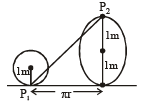Linear distance moved by the wheel in half revolution = πr
Point P1 after half revolution reaches at P2 vertically 2m above the ground.
∴ Displacement P1P= (π2r2 + 22)1/2 = 2 + 4)1/2     [∵ r = 1m]

31 Year NEET Previous Year Questions: System of Particles & Rotational Motion - 1 - Question 3

### If the linear density (mass per unit length) of a rod of length 3m is proportional to x, where x is the distance from one end of the rod, the distance of the centre of gravity of the rod from this end is: 

Detailed Solution for 31 Year NEET Previous Year Questions: System of Particles & Rotational Motion - 1 - Question 3

Consider an element of length dx at a distance x from end A.
Here mass per unit length λ of rod is:

λ ∝ x ⇒ λ = kx
∴ dm = λdx = kx dx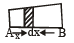Position of centre of gravity of rod from end A is: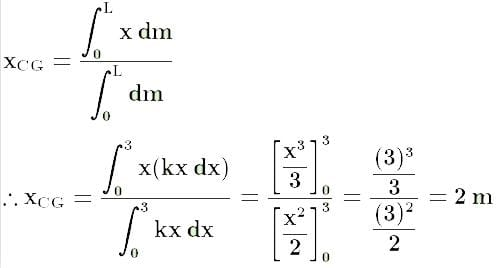31 Year NEET Previous Year Questions: System of Particles & Rotational Motion - 1 - Question 4

A disc is rotating with angular velocity ω. If a child sits on it, what is conserved?


Detailed Solution for 31 Year NEET Previous Year Questions: System of Particles & Rotational Motion - 1 - Question 4

If external torque is zero, angular momentum remains conserved (External torque is zero because the weight of the child acts downward).

L = Iω = constant

31 Year NEET Previous Year Questions: System of Particles & Rotational Motion - 1 - Question 5

A composite disc is to be made using equal masses of aluminium and iron so that it has as high a moment of inertia as possible. This is possible when:


Detailed Solution for 31 Year NEET Previous Year Questions: System of Particles & Rotational Motion - 1 - Question 5

Density of iron > Density of aluminium
Moment of inertia = ∫ r2 dm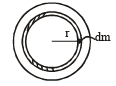Since, ρiron > ρaluminium
So, the whole of aluminium is kept in the core and the iron at the outer rim of the disc.

31 Year NEET Previous Year Questions: System of Particles & Rotational Motion - 1 - Question 6

When a mass is rotating in a plane about a fixed point, its angular momentum is directed along:


Detailed Solution for 31 Year NEET Previous Year Questions: System of Particles & Rotational Motion - 1 - Question 6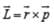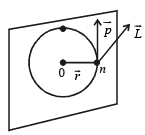• By right-hand screw rule, the direction of L is ⊥ to the plane containing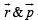.
• The mass is rotating in the plane, about a fixed point, thus this plane will containand the direction of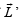will be perpendicular to this plane.
31 Year NEET Previous Year Questions: System of Particles & Rotational Motion - 1 - Question 7

Two persons of masses 55 kg and 65 kg respectively, are at the opposite ends of a boat. The length of the boat is 3.0 m and weighs 100 kg. The 55 kg man walks up to the 65 kg man and sits with him. If the boat is in still water the centre of mass of the system shifts by:


Detailed Solution for 31 Year NEET Previous Year Questions: System of Particles & Rotational Motion - 1 - Question 7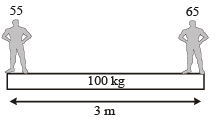There is no external force so centre of mass of the system will not shift.

31 Year NEET Previous Year Questions: System of Particles & Rotational Motion - 1 - Question 8

ABC is an equilateral triangle with O as its centre.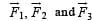represent three forces acting along the sides AB, BC and AC respectively. If the total torque about O is zero the magnitude of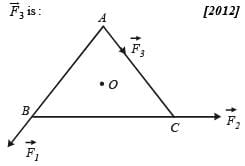Detailed Solution for 31 Year NEET Previous Year Questions: System of Particles & Rotational Motion - 1 - Question 8

The torques F1​d and F2​d of F1​ and F2​respectively are counterclockwise. The torque F3​d is clockwise. Applying condition for rotational equilibrium,

F1 d + F2 d = F3 d
F3 = F1 + F2

31 Year NEET Previous Year Questions: System of Particles & Rotational Motion - 1 - Question 9

A circular platform is mounted on a frictionless vertical axle. Its radius R = 2 m and its moment of inertia about the axle is 200 kgm2. It is initially at rest. A 50 kg man stands on the edge of the platform and begins to walk along the edge at the speed of 1 ms–1 relative to the ground. Time taken by the man to complete one revolution is:

Detailed Solution for 31 Year NEET Previous Year Questions: System of Particles & Rotational Motion - 1 - Question 9

By applying the law of conservation of angular momentum,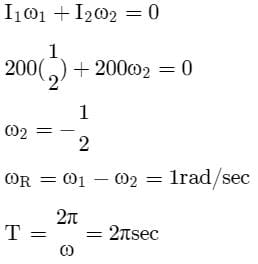31 Year NEET Previous Year Questions: System of Particles & Rotational Motion - 1 - Question 10

The moment of inertia of a uniform circular disc is maximum about an axis perpendicular to the disc and passing through:
[2012M]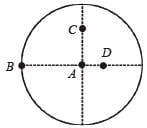Detailed Solution for 31 Year NEET Previous Year Questions: System of Particles & Rotational Motion - 1 - Question 10

According to the parallel axis theorem of the moment of Inertia,

I = Icm + md2

So, d is maximum for point B so Imax about B.

31 Year NEET Previous Year Questions: System of Particles & Rotational Motion - 1 - Question 11

Three masses are placed on the x-axis: 300g at origin, 500g at x = 40cm and 400g at x = 70 cm.The distance of the centre of mass from the origin is:
[2012M]

Detailed Solution for 31 Year NEET Previous Year Questions: System of Particles & Rotational Motion - 1 - Question 11

► Xcm = (m1x1 + m2x2 + m3x3) / (m1 + m2 + m3)
► Xcm = (300 x (0) + 500(40) + 400 x 70) / (300 + 500 + 400)
► Xcm = 500 x 40 + 400 x 70 / 1200
► Xcm = 50 + 70 / 3 = 120 / 3 = 40 cm

31 Year NEET Previous Year Questions: System of Particles & Rotational Motion - 1 - Question 12

A small object of uniform density rolls up a curved surface with an initial velocity  ‘ν’. It reaches up to a maximum height of 3v2 / 4g with respect to the initial position. The object is a:


Detailed Solution for 31 Year NEET Previous Year Questions: System of Particles & Rotational Motion - 1 - Question 12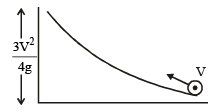From law of conservation of mechanical energy:
► 1 / 2 Iω2 + 0 + 1 / 2 mv2 = mg x 3v2 / 4g
► 1 / 2 Iω2 = 3 / 4 mv2 - 1 / 2 mv2
► 1 / 2 Iω= mv2 / 4
► (1 / 2 )* I * (V2 / R2) = mv2 / 4
► I = 1 / 2 mR2
Hence, object is a disc.

31 Year NEET Previous Year Questions: System of Particles & Rotational Motion - 1 - Question 13

A rod PQ of mass M and length L is hinged at end P. The rod is kept horizontal by a massless string tied to point Q as shown in the figure. When the string is cut, the initial angular acceleration of the rod is: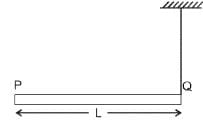Detailed Solution for 31 Year NEET Previous Year Questions: System of Particles & Rotational Motion - 1 - Question 13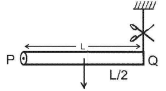Weight of the rod will produce the torque,
τ = mg x L / 2 = I α = mL2 / 3 α (∵ Irod = ML2 / 3)
Hence, angular acceleration, α = 3g / 2L

31 Year NEET Previous Year Questions: System of Particles & Rotational Motion - 1 - Question 14

Two discs are rotating about their axes, normal to the discs and passing through the centres of the discs. Disc D1 has 2 kg mass and 0.2 m radius and initial angular velocity of  50 rad s–1. Disc D2 has 4kg mass, 0.1 m radius and initial angular velocity of 200 rad s–1. The two discs are brought in contact face to face, with their axes of rotation coincident. The final angular velocity (in rad s–1) of the system is:


Detailed Solution for 31 Year NEET Previous Year Questions: System of Particles & Rotational Motion - 1 - Question 14

Given: m1 = 2 kg, m2 = 4 kg, r1 = 0.2 m, r2 = 0.1 m, w1 = 50 rad s–1, w2 = 200 rad s–1
As, I1W1 = I2W2 = Constant
∴ Wf = I1W1 + I2W2 / I1 + I2
= [(1 / 2 m1r1w)+ (1 / 2 m2r2w2 )] / (1 / 2 m1r12 + 1 / 2 m2r22)​
By putting the value of m1, m2, r1, r2 and solving we get final angular velocity = 100 rad s–1

31 Year NEET Previous Year Questions: System of Particles & Rotational Motion - 1 - Question 15

The ratio of radii of gyration of a circular ring and a circular disc, of the same mass and radius, about an axis passing through their centres and perpendicular to their planes are:


Detailed Solution for 31 Year NEET Previous Year Questions: System of Particles & Rotational Motion - 1 - Question 15

I = MK2  ⇒ K = √I / M
Iring = MR2 and Idisc = 1 / 2 MR2
► K1 / K2 = (I1 / I2 )1/2 = √MR2 / (MR2 / 2) = √2 : 1

## Physics Class 11

127 videos|464 docs|210 tests
 Use Code STAYHOME200 and get INR 200 additional OFF Use Coupon Code
Information about 31 Year NEET Previous Year Questions: System of Particles & Rotational Motion - 1 Page
In this test you can find the Exam questions for 31 Year NEET Previous Year Questions: System of Particles & Rotational Motion - 1 solved & explained in the simplest way possible. Besides giving Questions and answers for 31 Year NEET Previous Year Questions: System of Particles & Rotational Motion - 1, EduRev gives you an ample number of Online tests for practice

## Physics Class 11

127 videos|464 docs|210 tests

### How to Prepare for NEET

Read our guide to prepare for NEET which is created by Toppers & the best Teachers